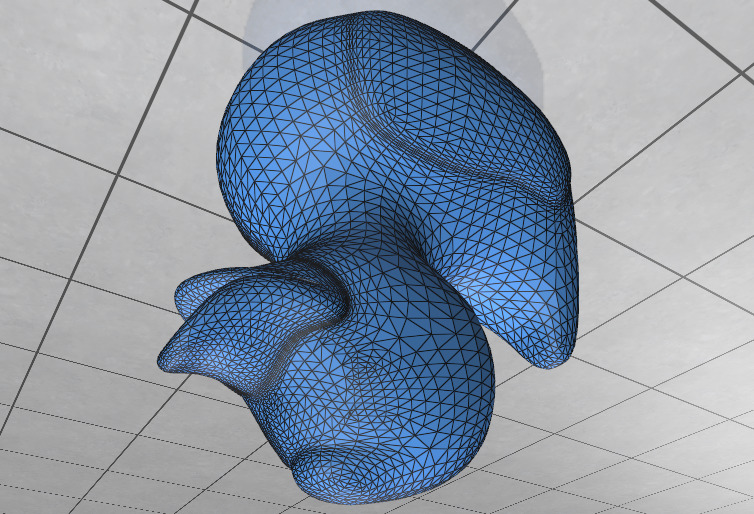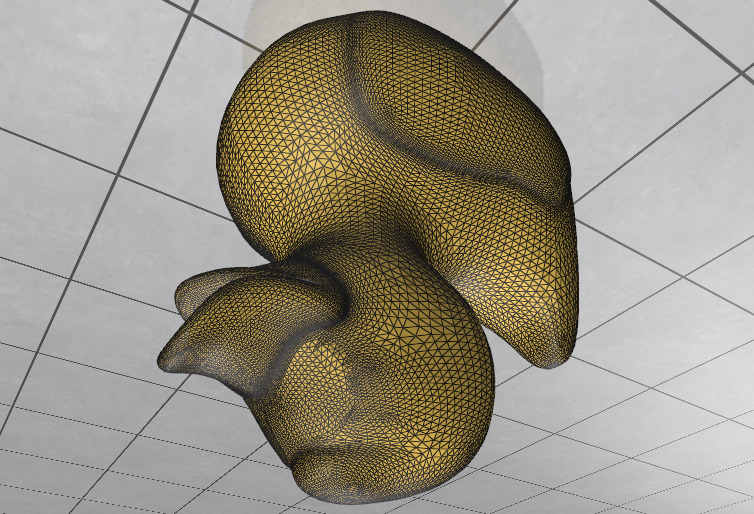# Basic Mutation

This tutorial explores the surface mesh data structure in geometry-central, via a simple example which splits and flips edges to perform 4:1 subidivision of a triangle mesh.

View full, runnable source code in the tutorial repository.

### Basic setup

To begin we include headers, bring in namespaces, See the first tutorial for more info on these steps.

#include "geometrycentral/surface/manifold_surface_mesh.h"
#include "geometrycentral/surface/meshio.h"
#include "geometrycentral/surface/surface_mesh.h"

#include "polyscope/polyscope.h"
#include "polyscope/surface_mesh.h"

using namespace geometrycentral;
using namespace geometrycentral::surface;


Again as before, load an input mesh from file, and visualize it using Polyscope. Here, we’ll require that the mesh be manifold.

std::unique_ptr<ManifoldSurfaceMesh> mesh;
std::unique_ptr<VertexPositionGeometry> geometry;

polyscope::init();
polyscope::registerSurfaceMesh("input mesh", geometry->vertexPositions,
mesh->getFaceVertexList());

// call polyscope::show(); to inspect the mesh at this point


Now, we’ll evaluate a 4:1 sudivision via the following algorithm:

• split each edge and connect to opposite vertices
• flip any new edge connecting a new to old vertex

First, we’ll create two MeshData<> containers. They associate data with mesh elements. We initialize them both with true values, because initially all vertices (resp. edges) are original. We’ll also track a list of edges to flip in the second phase.

VertexData<bool> isOrigVert(*mesh, true);
EdgeData<bool> isOrigEdge(*mesh, true);
std::vector<Edge> toFlip;


Now our main algorithm loop (commented inline).

for (Edge e : mesh->edges()) { // loop over all edges
if (!isOrigEdge[e]) continue; // don't keep processing new edges

// gather both vertices incident on the edge, and their positions
Vertex oldA = e.halfedge().tipVertex();
Vertex oldB = e.halfedge().tailVertex();
Vector3 oldAPos = geometry->vertexPositions[oldA];
Vector3 oldBPos = geometry->vertexPositions[oldB];

// split the edge
Vertex newV = mesh->splitEdgeTriangular(e).vertex();
isOrigVert[newV] = false;

// position the new vertex
Vector3 newPos = 0.5 * (oldAPos + oldBPos);
geometry->vertexPositions[newV] = newPos;

// iterate through the edges incident on the new vertex
for (Edge e : newV.adjacentEdges()) {
isOrigEdge[e] = false;                  // mark the new edges
Vertex otherV = e.otherVertex(newV);    // other side of edge

// if this is a new edge between an old an new vertex, save for flipping
if (isOrigVert[otherV] && otherV != oldA && otherV != oldB) {
toFlip.push_back(e);
}
}
}


and finally, we can actually flip the edges to complete the second phase

for (Edge e : toFlip) {
mesh->flip(e);
}


we now register this new mesh with geometry-central

auto* psMesh =
polyscope::registerSurfaceMesh("subdiv mesh",
geometry->vertexPositions,
mesh->getFaceVertexList());

polyscope::show();


and as expected get the subdivided mesh.

Before:After: